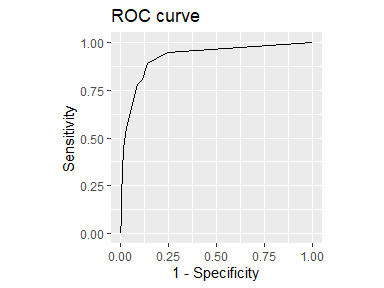# ROC curves with cutpointr

## Calculating only the ROC curve

When running cutpointr, a ROC curve is by default returned in the column roc_curve. This ROC curve can be plotted using plot_roc. Alternatively, if only the ROC curve is desired and no cutpoint needs to be calculated, the ROC curve can be created using roc() and plotted using plot_cutpointr. The roc function, unlike cutpointr, does not determine direction, pos_class or neg_class automatically.

library(cutpointr)
roc_curve <- roc(data = suicide, x = dsi, class = suicide,
pos_class = "yes", neg_class = "no", direction = ">=")
auc(roc_curve)
##  0.9237791
head(roc_curve)
## # A tibble: 6 x 9
##   x.sorted    tp    fp    tn    fn    tpr   tnr     fpr   fnr
##      <dbl> <dbl> <dbl> <int> <int>  <dbl> <dbl>   <dbl> <dbl>
## 1      Inf     0     0   496    36 0      1     0       1
## 2       11     1     0   496    35 0.0278 1     0       0.972
## 3       10     2     1   495    34 0.0556 0.998 0.00202 0.944
## 4        9     3     1   495    33 0.0833 0.998 0.00202 0.917
## 5        8     4     1   495    32 0.111  0.998 0.00202 0.889
## 6        7     7     1   495    29 0.194  0.998 0.00202 0.806
plot_roc(roc_curve)## ROC curve and optimal cutpoint for multiple variables

Alternatively, we can map the standard evaluation version cutpointr to the column names. If direction and / or pos_class and neg_class are unspecified, these parameters will automatically be determined by cutpointr so that the AUC values for all variables will be $$> 0.5$$.

We could do this manually, e.g. using purrr::map, but to make this task more convenient multi_cutpointr can be used to achieve the same result. It maps multiple predictor columns to cutpointr, by default all numeric columns except for the class column.

mcp <- multi_cutpointr(suicide, class = suicide, pos_class = "yes",
use_midpoints = TRUE, silent = TRUE)
summary(mcp)
## Method: maximize_metric
## Predictor: age, dsi
## Outcome: suicide
##
## Predictor: age
## --------------------------------------------------------------------------------
##  direction    AUC   n n_pos n_neg
##         <= 0.5257 532    36   496
##
##  optimal_cutpoint sum_sens_spec    acc sensitivity specificity tp fn  fp tn
##              55.5        1.1154 0.1992      0.9722      0.1431 35  1 425 71
##
## Predictor summary:
##     Data Min. 5% 1st Qu. Median    Mean 3rd Qu.   95% Max.      SD NAs
##  Overall   18 19      24   28.0 34.1259   41.25 65.00   83 15.0542   0
##       no   18 19      24   28.0 34.2218   41.25 65.50   83 15.1857   0
##      yes   18 18      22   27.5 32.8056   41.25 54.25   69 13.2273   0
##
## Predictor: dsi
## --------------------------------------------------------------------------------
##  direction    AUC   n n_pos n_neg
##         >= 0.9238 532    36   496
##
##  optimal_cutpoint sum_sens_spec    acc sensitivity specificity tp fn fp  tn
##               1.5        1.7518 0.8647      0.8889      0.8629 32  4 68 428
##
## Predictor summary:
##     Data Min.   5% 1st Qu. Median   Mean 3rd Qu.  95% Max.     SD NAs
##  Overall    0 0.00       0      0 0.9211       1 5.00   11 1.8527   0
##       no    0 0.00       0      0 0.6331       0 4.00   10 1.4122   0
##      yes    0 0.75       4      5 4.8889       6 9.25   11 2.5498   0

## Accessing data, roc_curve, and boot

The object returned by cutpointr is of the classes cutpointr, tbl_df, tbl, and data.frame. Thus, it can be handled like a usual data frame. The columns data, roc_curve, and boot consist of nested data frames, which means that these are list columns whose elements are data frames. They can either be accessed using [ or by using functions from the tidyverse. If subgroups were given, the output contains one row per subgroup and the function that accesses the data should be mapped to every row or the data should be grouped by subgroup.

set.seed(123)
opt_cut_b_g <- cutpointr(suicide, dsi, suicide, gender, boot_runs = 500)
library(dplyr)
library(tidyr)
opt_cut_b_g |>
group_by(subgroup) |>
select(subgroup, boot) |>
unnest(cols = boot) |>
summarise(sd_oc_boot = sd(optimal_cutpoint),
m_oc_boot  = mean(optimal_cutpoint),
m_acc_oob  = mean(acc_oob))
## # A tibble: 2 x 4
##   subgroup sd_oc_boot m_oc_boot m_acc_oob
##   <chr>         <dbl>     <dbl>     <dbl>
## 1 female        0.766      2.17     0.880
## 2 male          1.51       2.92     0.806

## Adding metrics to the result of cutpointr() or roc()

By default, the output of cutpointr includes the optimized metric and several other metrics. The add_metric function adds further metrics. Here, we’re adding the negative predictive value (NPV) and the positive predictive value (PPV) at the optimal cutpoint per subgroup:

cutpointr(suicide, dsi, suicide, gender, metric = youden, silent = TRUE) |>
select(subgroup, optimal_cutpoint, youden, ppv, npv)
## # A tibble: 2 x 5
##   subgroup optimal_cutpoint   youden      ppv      npv
##   <chr>               <dbl>    <dbl>    <dbl>    <dbl>
## 1 female                  2 0.808118 0.367647 0.993827
## 2 male                    3 0.625106 0.259259 0.982301

In the same fashion, additional metric columns can be added to a roc_cutpointr object:

roc(data = suicide, x = dsi, class = suicide, pos_class = "yes",
neg_class = "no", direction = ">=") |>
head()
## # A tibble: 6 x 7
## 6        7     7     1   495    29       0.301    0.318